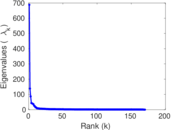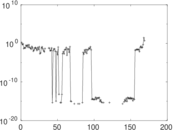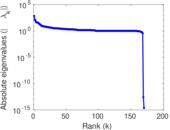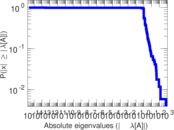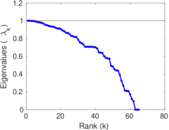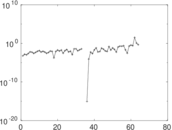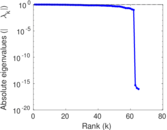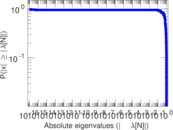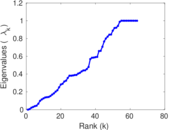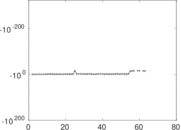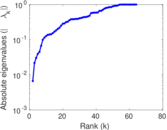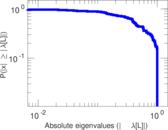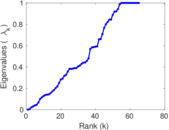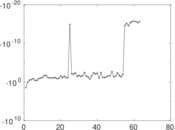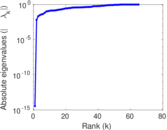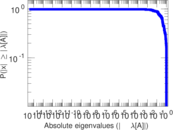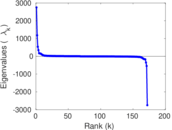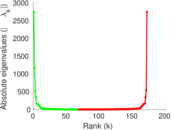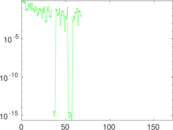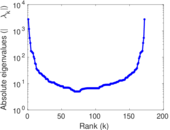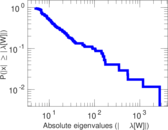# Wikibooks edits (km)

This is the bipartite edit network of the Khmer Wikibooks. It contains users and pages from the Khmer Wikibooks, connected by edit events. Each edge represents an edit. The dataset includes the timestamp of each edit.

 Code `bkm` Internal name `edit-kmwikibooks` Name Wikibooks edits (km) Data source http://dumps.wikimedia.org/ AvailabilityDataset is available for download Consistency checkDataset passed all tests Category Authorship network Dataset timestamp 2017-10-20 Node meaning User, article Edge meaning Edit Network formatBipartite, undirected Edge typeUnweighted, multiple edges Temporal dataEdges are annotated with timestamps

## Statistics

 Size n = 1,189 Left size n1 = 172 Right size n2 = 1,017 Volume m = 3,661 Unique edge count m̿ = 1,150 Wedge count s = 52,693 Claw count z = 3,299,865 Cross count x = 174,582,199 Square count q = 1,345 4-Tour count T4 = 223,876 Maximum degree dmax = 1,007 Maximum left degree d1max = 1,007 Maximum right degree d2max = 636 Average degree d = 6.158 12 Average left degree d1 = 21.284 9 Average right degree d2 = 3.599 80 Fill p = 0.006 574 28 Average edge multiplicity m̃ = 3.183 48 Size of LCC N = 821 Diameter δ = 14 50-Percentile effective diameter δ0.5 = 4.743 71 90-Percentile effective diameter δ0.9 = 7.733 73 Median distance δM = 5 Mean distance δm = 5.244 69 Gini coefficient G = 0.797 721 Balanced inequality ratio P = 0.172 904 Left balanced inequality ratio P1 = 0.107 894 Right balanced inequality ratio P2 = 0.233 270 Relative edge distribution entropy Her = 0.834 122 Power law exponent γ = 5.488 07 Tail power law exponent γt = 2.721 00 Tail power law exponent with p γ3 = 2.721 00 p-value p = 0.001 000 00 Left tail power law exponent with p γ3,1 = 1.841 00 Left p-value p1 = 0.429 000 Right tail power law exponent with p γ3,2 = 3.251 00 Right p-value p2 = 0.001 000 00 Degree assortativity ρ = −0.210 132 Degree assortativity p-value pρ = 6.078 83 × 10−13 Spectral norm α = 687.812 Algebraic connectivity a = 0.006 646 40 Spectral separation |λ1[A] / λ2[A]| = 4.974 39 Controllability C = 779 Relative controllability Cr = 0.698 655

## Plots

### Fruchterman–Reingold graph drawing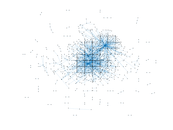### Degree distribution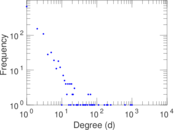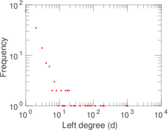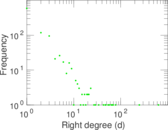### Cumulative degree distribution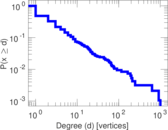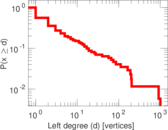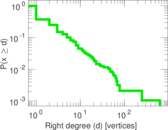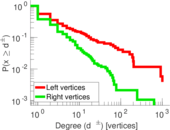### Lorenz curve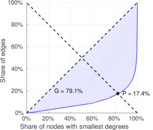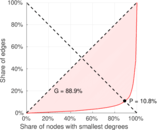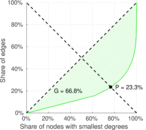### Spectral distribution of the adjacency matrix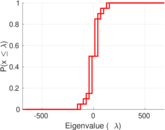### Spectral distribution of the normalized adjacency matrix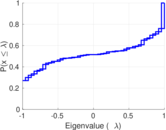### Spectral distribution of the Laplacian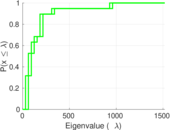### Spectral graph drawing based on the adjacency matrix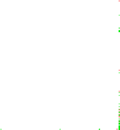### Spectral graph drawing based on the Laplacian### Spectral graph drawing based on the normalized adjacency matrix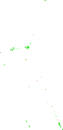### Degree assortativity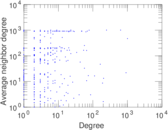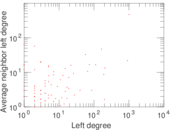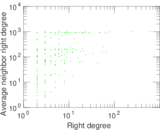### Zipf plot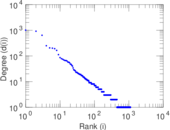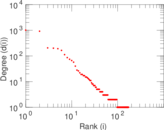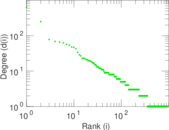### Hop distribution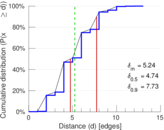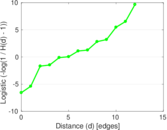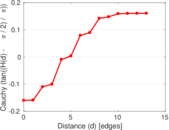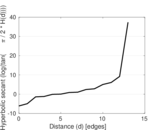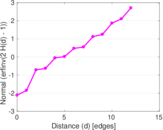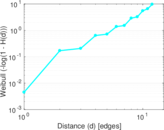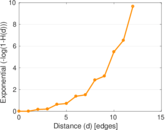### Double Laplacian graph drawing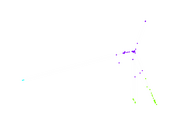### Delaunay graph drawing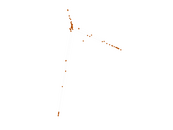### Edge weight/multiplicity distribution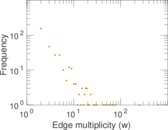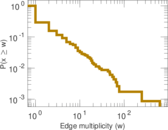### Temporal distribution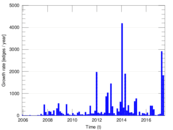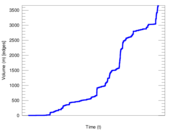### Temporal hop distribution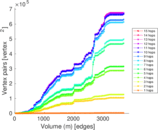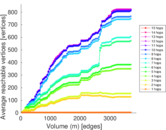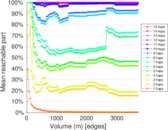### Diameter/density evolution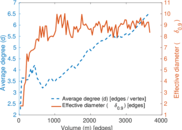### Matrix decompositions plots